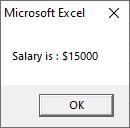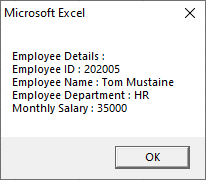# VBA VLookup

The VLookup is a worksheet function in excel, but we can also be used in the VBA. The functionality of the VLookup is similar to the feature in VBA and worksheet both.

In Excel, the VLookup function is used to search a value in an array and returns its corresponding value from another column. The searching value should be present in the first column.

The VLookup worksheet functions can be used in the VBA coding. The function can only call by using the worksheet, not built in the VBA.

### Syntax

The VLookup function follows the following syntax in Excel VBA:

Or

• Lookup_value is the value we are searching for.
• Table_array is the range we are searching through.
• Col_index_num is the column number of the return value.
• Range_lookup tells whether the match is exact or approximate. It can be 0/1 or true/false, use false to find the exact match. Use true to assume that the first column is sorted numerically or alphabetically.

## How to Use VLookup in Excel VBA

Step 1: Click on the Developer tab and choose the Visual Basic option.Step 2: Click on the Insert button and add a Module.Step 3: Then Write the VLookup program.

Step 4: Now run the code by using the Run button.

Let see calling the VLookup function in Excel VBA with the help of some examples that are given below:

### Examples

Suppose we have the data on a monthly salary of employees. The below table is displaying the salary of employees using a dialog box.

In the below table, the data is given in columns B and C. Employees ID in A cell, Name of employees in B cell, and the salary of the employees is returned in the C cell.Now write the VLookup code. And define the range in which data are present, i.e., column A, B, and C.

Define employee name as cell B2 and salary as cell C11. Now call the VLookup function using WorksheetFunction and put it in Sal.In the above example, we used a variable “E_name” to store the employee name whose salary is to be fetched. Gives the employee name to the VLookup, and it returns the salary of that employee.

Now execute the code using the Run button. And we will get the output shown in the below screenshot.Example 2: Here, we add a new column that is Department in the above table.Now write the VLookup code. And define the range in which data are present, i.e., column A, B, C, and D.

Write a code to call the VLookup function by using WorksheetFunction based on the Employee ID.

In the above example, we asked the user to enter the employee id, and then we used multiple VLookup statements and all the details in a single message box.

Run the above code using the Run button, enter the employee ID, which you want to enter, and click on the OK button.After clicking the OK button, we will get the output shown in the below screenshot.Next TopicVBA Date Format

Previous articleVBA Data Types
Next articleCircular reference in Excel